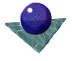Boundary formation on the
basis of local continuityIt is assumed that an edge detector, such as the Sobel or Canny operator, has been applied to an intensity image, although the processes described are equally applicable to 3D boundary data. Although not essential, it is easier to process data which has also been thresholded and thinned.

In many treatments, the objective is to create a continuous edge from a point p1 at ( x1 , y1 ) to another point p2 at ( x2 , y2 ) by finding the optimum path through the edge data. The basic principle is to form a graph from p1 to p2 in which the nodes represent the edge pixels and the arcs the one pixel steps between them in which each arc has an associated cost function. The cost function may be based on several criteria, but in general it is reasonable to install some function of the edge strength of the added pixel, the difference in edge direction between successive pixels and possibly to favour those pixels which are nearest to the direct line of sight to the final pixel, p2. Then the A* algorithm can be applied; the total cost function f( pi ) at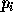is the sum of the path cost between the starting pixel p1 and the current pixel,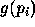, and an estimate of the path cost fromto the end pixel,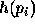.

```Expand the start node, n1  corresponding to p1 ; i.e. put all
successor nodes on a list called OPEN. Evaluate the cost
function of each expanded node.
While (OPEN is not empty) and (no solution output)
Remove the nodeof minimum cost function from OPEN.
If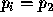then output the solution by tracing nodes
back to start node
else expand nodeby putting successors on OPEN and
evaluate the cost function of each expanded node.
```

This approach is fine, but the common problem is that the end pixel is not known when you start to track the boundary. Indeed the start pixel is not generally known either. Hence a yet more heuristic strategy must be adopted. Considering Figure 1, below, we wish to track firstly in front of the edge, i.e. in the direction b,c, or e, and then behind the edge, i.e. in the direction d,f or g. A possible algorithm is

```
Repeat

Scan the image to find the strongest remaining edge point.
Mark the edge as the initial and current contour point.
Repeat
Expand in front of the current contour point by adding the
unmarked edge point with the minimum cost function (as above).
Mark this latter point as the current point.
Until (no more edge points are found)
Mark the initial point as the current point
Repeat
Expand all unmarked edges behind the current contour point by
adding the unmarked edge point with the minimum cost
function (as above). Mark this latter point as the current point.
Until (no more edge points are found)
Save the list of marked edge points as a single contour
Until (no more edge points are found)
```Figure 1: Bidirectional edge tracking

Figure 1.1, below, shows an example of a local edge tracker, in this case applied to track contours on Ordnance survey maps as part of a wider project to create 3D models of terrain directly form scanned maps. In this case the cost function was based not only on edge strength and angle continuity, but also colour, i.e. it tracked the height contours which were brown, but ignored the river contours, for example, which were blue. Some of the associated problems are evident caused in the grid lines and lettering. In practice the Ordnance Survey maps are produced in layers, which makes the problem much easier as the raw data consists of only the contours. Nevertheless, commercial systems are not 100 per cent effective and some hand tracing is still required in most cases. The data in Figure 1.1 has additional post-processing of the tracked contours to allow bridging of small gaps.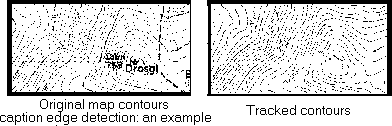The principal problem of an edge tracker based on local continuity of edge strength and direction, such as those described above, is the lack of the ability to encompass a wider area in making decisions. Thus, in many complex cases, the edge tracker may follow a locally stronger path caused for example by a shadow or specular highlight, at the expense of the ``true'' boundary. For this reason, they are usually applied to images which have very distinct isolated boundaries. The advantage of the approach is that no assumptions need be made a priori about the boundary shape, which may vary from a straight line to much more complex shapes which are difficult to parameterise.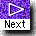[ Association of edge elements | Feature space transformation for line detection ]Comments to: Sarah Price at ICBL.
(Last update: 22th April, 1996)